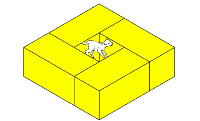## Tuesday, 8 August 2017

### Making Connections

If  algebra is about seeing patterns and making connections, how can we connect number, geometry & algebra?

One approach... think about the number 180;
• 180 degrees on a straight line
• 180 degrees is the sum of the interior angles in a triangle
• 180 degrees in a semicircle
• 180 is an even number and a multiple of 10
• 180 in standard form looks like this 1.8 x 10 ^2

Is 180 a happy or an unhappy number?

1. What pairs of numbers add up to 180?
2. How many different pairs can you find?
3. What patterns can you see?
4. How do you know if you have found all the pairs?

A spreadsheet is one way for students to experiment :

Use the View> Show Formula option to introduce students to the algebraic logic behind spreadsheet formulae

How could we draw this?
How else could we draw this?

How could this be written using symbols?
120 + 60
or 120 + 60 = 180

Target 180
Two numbers add to 180. If I know one number what could the other number be?
Use a table like this as a starter/energiser  for a lesson

How else could this idea be represented ?

A picture maybe?

Using symbols and text?
120 + ? = target   or 120 + x = target

In this case the target is 180 so we could write :
120 + x = 180

What would this equation look like if the target number changed?
What does this tell us about the values that x can take?Other ideas might involve rectangles , perimeters,  area

What other ways can we connect algebra, geometry & number?